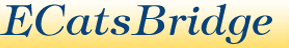"Promoting Real Bridge in a Virtual World" - Developed by Anna Gudge and Mark NewtonWorld Events:

Neuberg Formula

If the boards in an event haven't all been played the same number of times the Neuberg formua is applied. This formula states:

 N S = (s+1) - 1 n

where:

• N = normal number of results
• n = actual number of results
• s = score according to normal calculation

S is then the factored score